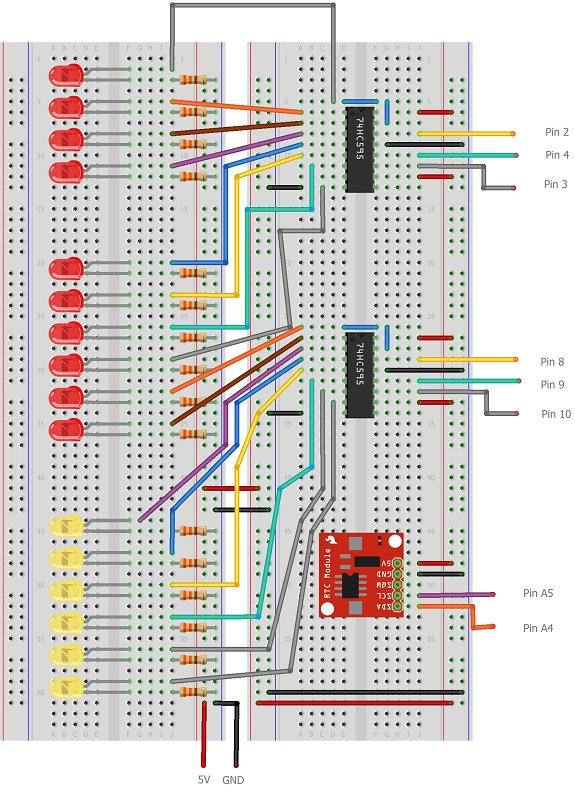# Binary Clock

## Introduction

In this project, 16 LEDs are used to represent the time in binary. The time is read from a Real Time Clock breakout board – the same one used in the Digital Clock page.The binary clock uses 4 place values for the hour (12hr format), 6 place values for the minutes, and 6 for the seconds.So the following pattern…… makes the time, 8+2 = 10 hours, 16 + 4 + 1 = 21 minutes, 4+2+1 = 7 seconds.

## You Will Need

• DS1307 Breakout Board & RTC
• 2 x 74HC595 Shift Registers
• 16 x LEDs
• 16 x 330 Ohm Resistors
• Jumper Wires

## Making The Circuit

Take care with this one, there are a lot of things to get connected. The Arduino isn’t shown in this diagram. The text labels show the pin connections – the connections to the shift registers are to digital pins.

Here is a blurry photograph of the binary clock circuit set up on an Arduino Mega. There are some minor differences to the diagram to account for the differences between the Uno and the Mega. Some of the components (eg resistors) are also slightly different. However, it works just like the one in the diagram.

## Programming The Arduino

The tricky part of this code is to create the correct binary pattern. The first shift register controls the 4 LEDs that make the hours as well as 4 of the LEDs that make up the minutes. The second shift register does the other 2 LEDs of the minutes as well as all of the seconds.Because of the number of places where a faulty or missing connection can prevent this from working, it’s worth just checking that you can light all of the LEDs properly. With the rest of the code as below, replace the loop with the following lines to make sure that all of the LEDs can be lit,

READ  Binary metric clock using Arduino

```  writeByte(255,1); writeByte(255,2);```

The main program,

```#include <Wire.h> #include "RTClib.h"```

``` RTC_DS1307 RTC; int datapin = 2; int clockpin = 3; int latchpin = 4; int datapin2 = 8; int clockpin2 = 9; int latchpin2= 10; void setup() { Serial.begin(57600); Wire.begin(); RTC.begin(); if (! RTC.isrunning()) { Serial.println("RTC is NOT running!"); // following line sets the RTC to the date & time this sketch was compiled //RTC.adjust(DateTime(__DATE__, __TIME__)); } pinMode(datapin, OUTPUT); pinMode(clockpin, OUTPUT); pinMode(latchpin, OUTPUT); pinMode(datapin2, OUTPUT); pinMode(clockpin2, OUTPUT); pinMode(latchpin2, OUTPUT); } void loop() { DateTime now = RTC.now(); // All used for checking the time of the clock // This section can be removed when everything is working Serial.print(now.day(), DEC); Serial.print('/'); Serial.print(now.month(), DEC); Serial.print('/'); Serial.print(now.year(), DEC); Serial.print(' '); Serial.print(now.hour(), DEC); Serial.print(':'); Serial.print(now.minute(), DEC); Serial.print(':'); Serial.print(now.second(), DEC); Serial.println(); // End of section that can be removed int mins = now.minute(); int secs = now.second(); int hr = now.hour(); // convert to 12 hour time if (hr>12) { hr = hr-12; } // variables to describe pattern of on lights byte data1 = 0; byte data2 = 0; // encode the time // hr = 1st four bits for (int i =0;i<4;i++) { if (bitRead(hr,i)==1) { bitWrite(data1,3-i,1); } } // mins on the first shift register for (int i =2;i<6;i++) { if (bitRead(mins,i)==1) { bitWrite(data1,9-i,1); } } // remaining mins LEDs for (int i =0;i<2;i++) { if (bitRead(mins,i)==1) { bitWrite(data2,1-i,1); } } // seconds for (int i =2;i<8;i++) { if (bitRead(secs,i-2)==1) { bitWrite(data2,9-i,1); } } // output the information writeByte(data1,1); writeByte(data2,2); // a brief pause delay(500); } ```

```void writeByte(byte data, byte set) { int d,c,l; if (set==1) { d = 2; c = 3; l = 4; } else if (set==2) { d = 8; c = 9; l = 10; } shiftOut(d, c, MSBFIRST, data); // toggle the latch pin so that the data appears as an output digitalWrite(l, HIGH); digitalWrite(l, LOW); } ```

For more detail: Binary Clock

#### This Post / Project can also be found using search terms:

• binary clock project
• binary clock setup
• arduino binary clock with rtc
• arduino uno binary clock